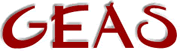[ < ] [ > ] [ << ] [ Up ] [ >> ] [Top] [Contents] [Index] [ ? ]

### 2.2.4 Type identification

Due to the fact that all variables are initialized to 0, and that many functions return 0 when failing, it's desirable to be able to determine what kind of value type you actually have received. Also, if you use the `mixed` type it's virtually essential to be able to test what the variable contains at times. For this purpose there's a special test function for each type that will return 1 (true) if the tested value is of the asked for type, and 0 if not.

@bullet{int intp(mixed)}
Test if given value is an integer

@bullet{int floatp(mixed)}
Test if given value is a float

@bullet{functionp(mixed)}
Test if given value is a function pointer

@bullet{int stringp(mixed)}
Test if given value is a string

@bullet{int objectp(mixed)}
Test if given value is an object pointer

@bullet{int mappingp(mixed)}
Test if given value is a mapping

@bullet{int pointerp(mixed)}
Test if given value is an array

NB! These functions test the type of the value, NOT the value itself in the sense of truth functionality. In other words `intp(0)` will always evaluate as true, as will `mappingp(([]))`.

This document was generated by Ronny Wikh on July, 8 2003 using texi2html